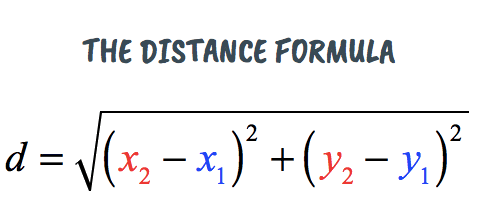Finding the hypotenuse
Distance Formula
Finding leg
Vocabulary

C√82-22

8.25

### 100

(-3,.2) (5,-3)?

≈8.616263691415206

B2=102-82

B=6

### 100

Are Pythagorean Theorem and Distance Formula the same?

Yes

C√5^2-9^2

10.3

(5,2) (1,2)

4

B2=62-112

B=9.2

### 200

hypotenuse

The longest side of a right triangle.

C√522-212

56.08

(4,2)(9,2)

5

B=52-132

12

### 300

legs

The sides of a right triangle that form a 90 degree angle.

C√242-312

39.1

### 400

(-3,-6) (2,9)

15.811388300841896

B2=82-152

12.69

### 400

Distance FormulaC√672-412

78.55

### 500

(-9,-12) (-2,9)

22.135943621178654

B2=252-302

16.58

### 500

right triangle

a triangle with one right angle